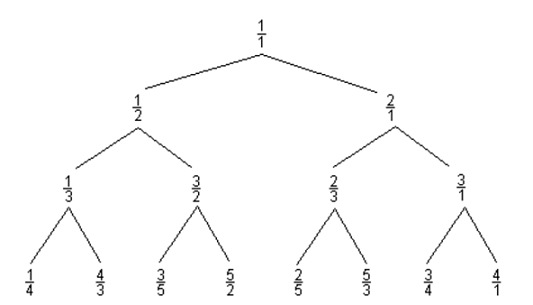# Perl Weekly Challenge 146: Curious Fraction Tree

by Abigail## Challenge

Consider the following Curious Fraction Tree:You are given a fraction, member of the tree created similar to the above sample.

Write a script to find out the parent and grandparent of the given member.

Input: $member = '3/5'; Output: parent = '3/2' and grandparent = '1/2'  ### Example 2 Input:$member = '4/3';
Output: parent = '1/3' and grandparent = '1/2'


## Discussion

This challenge is defined piss poorly. All we have is an image, a name, and to do something with a member of a tree create similar to the above example. We can only guess what the similar tree looks like. Does it start with a different root? Does the similar tree actually have more than 15 members? Is there a different relation between parent nodes and children nodes?

Let's just ignore everything past the first sentence, go to Google, and type in "Curious Fraction Tree", and just go whatever the first hit gives.

Hmmm, that's easy! A curious fraction tree is a tree with the following properties:

• The root contains 1/1
• If a node contains a/b, then its children contain a/(a + b) and (a + b)/b.

That's it! See how easy it is to write an unambiguous description? No need for participants to extrapolate from an image. No confusing references to similar trees.

Some people may call ambiguous challenges a feature. I call it sloppiness.

Oh, BTW, given the image above, what is the grandparent of 1/2? What's the parent of 1/1? Aargh. More sloppiness!

## Solution

We will be reading fractions from standard input, one fraction per line. For each read fraction, we'll write the parent and grandparent on a line of standard output. (If a fraction doesn't have a grandparent, we only write the parent; if the fraction doesn't have a parent, we write an empty line.)

Given the definition of the tree, given in the "Discussion" section above, it's really easy to calculate the parent $$\mathcal{P}$$ of a node:

$\mathcal{P} \left(\frac{a}{b}\right) = \begin{cases} \frac{a}{b - a} & \text{if } a < b \\ \frac{a - b}{b} & \text{if } a > b \end{cases}$

### Perl

Translating the formula above is easy. We just have to take care of not showing the root of 1/1. This happens if either the numerator or denominator of the calculation is 0. If so, we exit the loop early.

while (<>) {
my ($a,$b) = /[0-9]+/g;
for (1, 2) {
$a <$b ? ($b -=$a) : ($a -=$b);
last unless $a &&$b;
print "$a/$b ";
}
print "\n";
}


Find the full program on GitHub.

### Other languages

We also have solutions in the following languages:

AWK, Bash, bc, C, Go, Java, Lua, Node.js, Pascal, Python, R, Ruby, Tcl, and Scheme.

They all use the same algorithm as we used in the Perl solution.